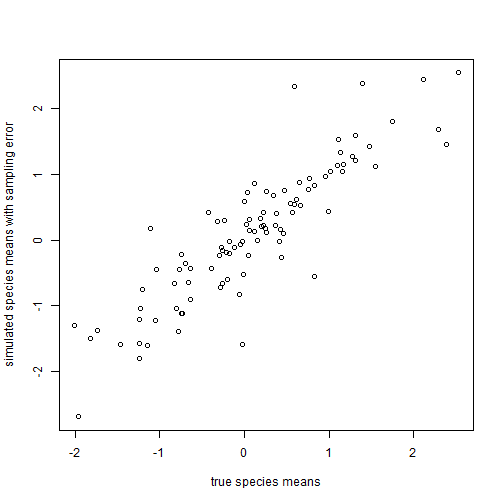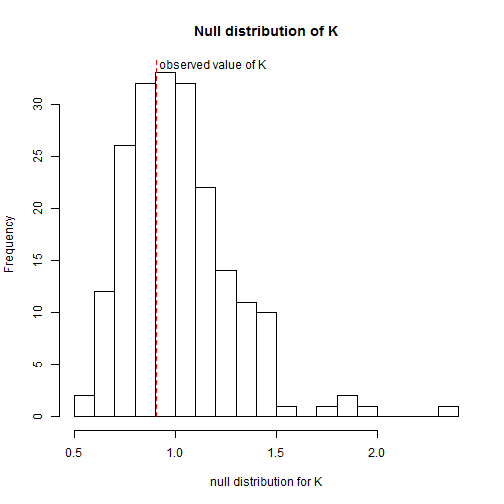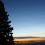## Thursday, June 11, 2015

### Testing a null hypothesis of K=1.0 with measurement error in the estimation of trait means for species

In the past I've described a “parametric bootstrapping” approach for testing the null hypothesis that Blomberg's K is significantly different from 1.0 (for instance here). 1.0 is the expected value of Blomberg's K under a Brownian motion model of evolutionary change for our character. Here, I'll describe the relatively simple modification of that procedure that can be used to conduct the same hypothesis test under conditions in which we also have sampling error in the estimation of species means for our character.

Let's start by simulating some trait data with sampling error. We can do this using the phytools functions `pbtree` (for phylogeny simulation), `fastBM` (to simulate trait data), and `sampleFrom`, which is a simple function, normally used internally, which allows us to sample trait data under some model (for instance, with error).

``````library(phytools)
## simulate tree
tree<-pbtree(n=100,scale=1)
## simulate trait data
x<-fastBM(tree,sig2=1)
ve<-setNames(rchisq(n=Ntip(tree),df=1)/runif(n=Ntip(tree),min=1,max=5),
tree\$tip.label)
xe<-sampleFrom(x,ve,n=rep(1,Ntip(tree)))
plot(x,xe,xlab="true species means",
ylab="simulated species means with sampling error")
``````Now let's fit our model & generate data under the null hypothesis of K = 1.0. This is where we would normally begin with a genuine empirical dataset.

``````fit<-phylosig(tree,xe,se=sqrt(ve))
fit
``````
``````## \$K
##  0.9085662
##
## \$sig2
##  1.125822
##
## \$logL
##  -112.5553
``````
``````nullX<-fastBM(tree,nsim=200,sig2=fit\$sig2)
nullXe<-apply(nullX,2,sampleFrom,xvar=ve,n=rep(1,Ntip(tree)))
obj<-apply(nullXe,2,phylosig,tree=tree,se=sqrt(ve))
nullK<-sapply(obj,function(x) x[])
hist(nullK,breaks=20,xlab="null distribution for K",
main="Null distribution of K")
lines(c(fit\$K,fit\$K),c(0,par()\$usr),lty="dashed",col="red")
text(x=fit\$K,y=0.985*par()\$usr,"observed value of K",
pos=4,offset=0.2)
``````Obviously, here we are well within the null distribution for K, but we can also attach a P-value to this observation. I usually use the logarithm of K, because this equally penalizes K = 0.5 and K = 2.0.

``````P<-mean(abs(log(nullK))>=abs(log(fit\$K))) ## two-tailed test
P
``````
``````##  0.675
``````

That's it.

#### 1 comment:

1.Hi Liam,

Thanks so much for providing this, and your previous post on testing against K=1. I am using this approach for the analysis in a manuscript I am working on. How should I cite you?

Note: due to the very large amount of spam, all comments are now automatically submitted for moderation.